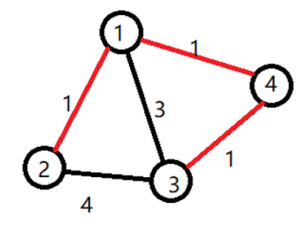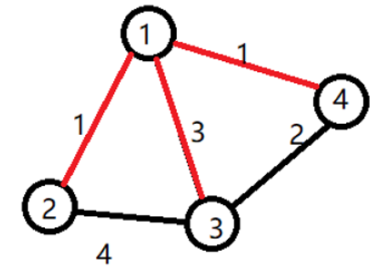# #333. 【NOIP2017】宝藏

### 样例一

#### input

4 5
1 2 1
1 3 3
1 4 1
2 3 4
3 4 1



#### output

4



#### explanation### 样例二

#### input

4 5
1 2 1
1 3 3
1 4 1
2 3 4
3 4 2



#### output

5



#### explanation### 限制与约定

• 对于20%的数据：
• 保证输入是一棵树，$1\le n\le 8$，$v\le 5000$ 且所有的 $v$ 都相等。
• 对于40%的数据：
• $1\le n\le 8$，$0\le m\le 1000$，$v\le 5000$ 且所有的 $v$ 都相等。
• 对于70%的数据：
• $1\le n\le 8$，$0\le m\le 1000$，$v\le 5000$
• 对于100%的数据：
• $1\le n\le 12$，$0\le m\le 1000$，$v\le 500000$